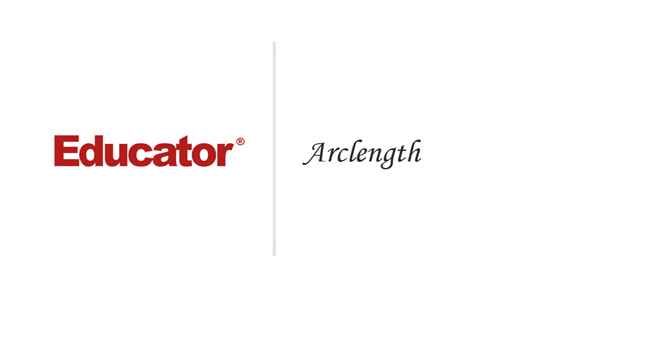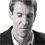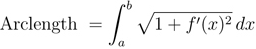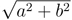Professor Murray

Arclength

Slide Duration:

Section 1: Advanced Integration Techniques
Integration by Parts

24m 52s

Intro
0:00
Important Equation
0:07
Where It Comes From (Product Rule)
0:20
Why Use It?
0:35
Lecture Example 1
1:24
Lecture Example 2
3:30
Shortcut: Tabular Integration
7:34
Example
7:52
Lecture Example 3
10:00
Mnemonic: LIATE
14:44
Ln, Inverse, Algebra, Trigonometry, e
15:38
-1
-2
Integration of Trigonometric Functions

25m 30s

Intro
0:00
Important Equation
0:07
Powers (Odd and Even)
0:19
What To Do
1:03
Lecture Example 1
1:37
Lecture Example 2
3:12
Half-Angle Formulas
6:16
Both Powers Even
6:31
Lecture Example 3
7:06
Lecture Example 4
10:59
-1
-2
Trigonometric Substitutions

30m 9s

Intro
0:00
Important Equations
0:06
How They Work
0:35
Example
1:45
Remember: du and dx
2:50
Lecture Example 1
3:43
Lecture Example 2
10:01
Lecture Example 3
12:04
-1
-2
Partial Fractions

41m 22s

Intro
0:00
Overview
0:07
Why Use It?
0:18
Lecture Example 1
1:21
Lecture Example 2
6:52
Lecture Example 3
13:28
-1
-2
Integration Tables

20m

Intro
0:00
Using Tables
0:09
Match Exactly
0:32
Lecture Example 1
1:16
Lecture Example 2
5:28
Lecture Example 3
8:51
-1
-2
Trapezoidal Rule, Midpoint Rule, Left/Right Endpoint Rule

22m 36s

Intro
0:00
Trapezoidal Rule
0:13
Graphical Representation
0:20
How They Work
1:08
Formula
1:47
Why a Trapezoid?
2:53
Lecture Example 1
5:10
Midpoint Rule
8:23
Why Midpoints?
8:56
Formula
9:37
Lecture Example 2
11:22
Left/Right Endpoint Rule
13:54
Left Endpoint
14:08
Right Endpoint
14:39
Lecture Example 3
15:32
-1
-2
Simpson's Rule

21m 8s

Intro
0:00
Important Equation
0:03
Estimating Area
0:28
Difference from Previous Methods
0:50
General Principle
1:09
Lecture Example 1
3:49
Lecture Example 2
6:32
Lecture Example 3
9:07
-1
-2
Improper Integration

44m 18s

Intro
0:00
Horizontal and Vertical Asymptotes
0:04
Example: Horizontal
0:16
Formal Notation
0:37
Example: Vertical
1:58
Formal Notation
2:29
Lecture Example 1
5:01
Lecture Example 2
7:41
Lecture Example 3
11:32
Lecture Example 4
15:49
Formulas to Remember
18:26
Improper Integrals
18:36
Lecture Example 5
21:34
Lecture Example 6 (Hidden Discontinuities)
26:51
-1
-2
Section 2: Applications of Integrals, part 2
Arclength

23m 20s

Intro
0:00
Important Equation
0:04
Why It Works
0:49
Common Mistake
1:21
Lecture Example 1
2:14
Lecture Example 2
6:26
Lecture Example 3
10:49
-1
-2
Surface Area of Revolution

28m 53s

Intro
0:00
Important Equation
0:05
Surface Area
0:38
Relation to Arclength
1:11
Lecture Example 1
1:46
Lecture Example 2
4:29
Lecture Example 3
9:34
-1
-2
Hydrostatic Pressure

24m 37s

Intro
0:00
Important Equation
0:09
Main Idea
0:12
Different Forces
0:45
Weight Density Constant
1:10
Variables (Depth and Width)
2:21
Lecture Example 1
3:28
-1
-2
Center of Mass

25m 39s

Intro
0:00
Important Equation
0:07
Main Idea
0:25
Centroid
1:00
Area
1:28
Lecture Example 1
1:44
Lecture Example 2
6:13
Lecture Example 3
10:04
-1
-2
Section 3: Parametric Functions
Parametric Curves

22m 26s

Intro
0:00
Important Equations
0:05
Slope of Tangent Line
0:30
Arc length
1:03
Lecture Example 1
1:40
Lecture Example 2
4:23
Lecture Example 3
8:38
-1
-2
Polar Coordinates

30m 59s

Intro
0:00
Important Equations
0:05
Polar Coordinates in Calculus
0:42
Area
0:58
Arc length
1:41
Lecture Example 1
2:14
Lecture Example 2
4:12
Lecture Example 3
10:06
-1
-2
Section 4: Sequences and Series
Sequences

31m 13s

Intro
0:00
Definition and Theorem
0:05
Monotonically Increasing
0:25
Monotonically Decreasing
0:40
Monotonic
0:48
Bounded
1:00
Theorem
1:11
Lecture Example 1
1:31
Lecture Example 2
11:06
Lecture Example 3
14:03
-1
-2
Series

31m 46s

Intro
0:00
Important Definitions
0:05
Sigma Notation
0:13
Sequence of Partial Sums
0:30
Converging to a Limit
1:49
Diverging to Infinite
2:20
Geometric Series
2:40
Common Ratio
2:47
Sum of a Geometric Series
3:09
Test for Divergence
5:11
Not for Convergence
6:06
Lecture Example 1
8:32
Lecture Example 2
10:25
Lecture Example 3
16:26
-1
-2
Integral Test

23m 26s

Intro
0:00
Important Theorem and Definition
0:05
Three Conditions
0:25
Converging and Diverging
0:51
P-Series
1:11
Lecture Example 1
2:19
Lecture Example 2
5:08
Lecture Example 3
6:38
-1
-2
Comparison Test

22m 44s

Intro
0:00
Important Tests
0:01
Comparison Test
0:22
Limit Comparison Test
1:05
Lecture Example 1
1:44
Lecture Example 2
3:52
Lecture Example 3
6:01
Lecture Example 4
10:04
-1
-2
Alternating Series

25m 26s

Intro
0:00
Main Theorems
0:05
Alternation Series Test (Leibniz)
0:11
How It Works
0:26
Two Conditions
0:46
Never Use for Divergence
1:12
Estimates of Sums
1:50
Lecture Example 1
3:19
Lecture Example 2
4:46
Lecture Example 3
6:28
-1
-2
Ratio Test and Root Test

33m 27s

Intro
0:00
Theorems and Definitions
0:06
Two Common Questions
0:17
Absolutely Convergent
0:45
Conditionally Convergent
1:18
Divergent
1:51
Missing Case
2:02
Ratio Test
3:07
Root Test
4:45
Lecture Example 1
5:46
Lecture Example 2
9:23
Lecture Example 3
13:13
-1
-2
Power Series

38m 36s

Intro
0:00
Main Definitions and Pattern
0:07
What Is The Point
0:22
Radius of Convergence Pattern
0:45
Interval of Convergence
2:42
Lecture Example 1
3:24
Lecture Example 2
10:55
Lecture Example 3
14:44
-1
-2
Section 5: Taylor and Maclaurin Series
Taylor Series and Maclaurin Series

30m 18s

Intro
0:00
Taylor and Maclaurin Series
0:08
Taylor Series
0:12
Maclaurin Series
0:59
Taylor Polynomial
1:20
Lecture Example 1
2:35
Lecture Example 2
6:51
Lecture Example 3
11:38
Lecture Example 4
17:29
-1
-2
Taylor Polynomial Applications

50m 50s

Intro
0:00
Main Formulas
0:06
Alternating Series Error Bound
0:28
Taylor's Remainder Theorem
1:18
Lecture Example 1
3:09
Lecture Example 2
9:08
Lecture Example 3
17:35
-1
-2
This is a quick preview of the lesson. For full access, please Log In or Sign up.
For more information, please see full course syllabus of College Calculus: Level II
Bookmark & Share Embed

## Copy & Paste this embed code into your website’s HTML

Please ensure that your website editor is in text mode when you paste the code.
(In Wordpress, the mode button is on the top right corner.)
×
• - Allow users to view the embedded video in full-size.

• ## Related Books1 answerLast reply by: Dr. William MurrayMon Mar 12, 2018 9:51 AMPost by kevin thompson on March 10, 2018Just to warn a few people; watch out, as I have never encountered a single problem where you can use these steps.  This is the original video I learned my steps off of and continued to do EVERY single question from there on out wrong.  99.99999% of questions require using U-sub and attacking the integral first.  You can absolutely ignore this example!  Example, try this using y=x^2/3 + 11 answerLast reply by: Dr. William MurrayFri Oct 4, 2013 6:53 PMPost by Narin Gopaul on September 29, 2013I am sorry i saw the mistake I maked1 answerLast reply by: Dr. William MurrayFri Oct 4, 2013 6:52 PMPost by Narin Gopaul on September 29, 2013I think you made a mistake in the first example by saying that u add 1/2 to the function i thought you would -1/21 answerLast reply by: Dr. William MurrayFri Dec 7, 2012 4:48 PMPost by Riley Argue on June 3, 2012Excellent lecture.You elegantly and simply explained this, thank you.3 answersLast reply by: Dr. William MurrayFri Dec 7, 2012 4:47 PMPost by Alphonse Mbu on March 7, 2012why do you use a^2+b^2-2ab. i dont understand what makes that valid

### Arclength

Main formula:Hints and tips:

• To remember this formula, it helps to recall that it comes from the distance formula between two points, which in turn comes from the Pythagorean Theorem.

• Remember that you must integrate the square root formula above. A common mistake is to integrate the function itself, not the square root formula. Of course, this would give you the area under the curve and not the arclength.

• A similar mistake is to mix this up with formula for surface area of revolution, which looks similar. Be careful which one you are asked for.

• Don’t make the common algebraic mistake of thinking thatreduces to a + b! This is extremely wrong, and your teacher will likely be merciless if you do it!

• Many problems in Calculus II classes are “rigged” so that when you expand 1 + f ′(x , it becomes a perfect square that cancels nicely with the square root.

• Often this perfect square is achieved by making the f ′(x be something of the form (a − b)² = a²− 2ab + b². Then the +1 changes it to a² + 2ab + b², which you can then factor as (a + b.

• When it’s feasible, check that your answer makes sense. Unlike area integrals, which can be negative if a curve goes below the x-axis, arclength should always be positive! You might also be able to check that the curve looks about as long as your answer.

### Arclength

Lecture Slides are screen-captured images of important points in the lecture. Students can download and print out these lecture slide images to do practice problems as well as take notes while watching the lecture.

• Intro 0:00
• Important Equation 0:04
• Why It Works
• Common Mistake
• Lecture Example 1 2:14
• Lecture Example 2 6:26
• Lecture Example 3 10:49
• Additional Example 4
• Additional Example 5

### Transcription: Arclength

We are here to look at a couple more examples of arc length problems.0000

The first one I have set up here is y = x4/8 + 1/4x2.0005

So remember with the arc length, you do not integrate it directly.0012

That is a common mistake that Calculus 2 students make is just integrating the function they are given.0015

When you want to find the arc length, you have got to find the derivative f'.0019

So here, the derivative of x4 is 4x3/8 is just x3/2.0026

Now, if you think of x2 in the denominator as x-2,0037

The derivative of that is x-2 x-3.0042

That is why I have a negative here - 2x-3,0048

Then because of that 4 in the denominator, the two and the four cancel and we get a 2 in the denominator.0052

x-3 gives you an x3 in the denominator.0060

So, that is what we get for the derivative.0066

We need to square that, f'(x)2 is x6/4 + 1/4x6, squaring out both terms.0069

Then, minus 2ab, so minus 2 × 1/2 × 1/2 is 1/2.0085

Then the x3 cancel, and this term is what we get by doing the -2ab of the a - b2 formula.0094

So, that is where that - 1/2 comes in.0107

1 + f'(x)2 is x6/4, still + 1/4x6.0109

Now, - 1/2 + 1 gives us + 1/2.0122

That is almost the same function that we had before except that the minus has turned into a plus.0130

What started out as a-b2, this is now going to turn into a+b2.0137

So this is x3/2 + 1/2x3 quantity squared.0144

Again, this is a very common pattern in arc length problems.0154

They often kind of rig up these examples so that the answer you get for 1 + f'(x)2 factors nicely into a perfect square.0158

It is sometimes not so obvious but if you look for it, it is often there.0168

That makes it nice because 1 + f'(x)2, when we take the square root will just simplify back down to x3/2 + 1/2x3.0173

So, the arc length is the integral of that function.0186

So that is the integral from x=1 to x=2, getting those values from the stem of the problem.0191

Of x3/2 + 1/2x3 dx.0199

If we integrate x3 that is x4/4, so this is x4/8.0210

Now, the integral of x-3 is x-2/-2, using the power rule.0217

So this is -, because the -2 in the denominator, 1/4x2, that is -2x-2.0229

Then there is another 2 coming from the 2 there.0240

So, we want a value like this from x=1 to x=2.0244

So, what we get is 24/8, that is 16/8, so that is just 2.0251

Minus 1/4 × 22, so that is 1/16.0257

Minus, now if you plug in x=1, you get 1/8.0266

Plus 1/4, so if we think of everything in terms of 16ths there,0270

That is 32 - 1 - 2 + 4/16.0277

So that simplifies down to 33/16.0287

As our final answer for the arc length0294

So the point there is that we take the function we are given, find its derivative, run it through this Pythagorean formula,0297

And we got lucky here in that the perfect square and the square roots cancel.0308

Then integrate the thing you get there.0315

Let us do 1 more example here, we want to find the arc length of y=ln(x)/2-x2/4.0000

So we do not integrate the function directly, we look at its derivative.0010

f' is, well, the derivative of ln(x) is 1/x so this is 1/2x - the derivative of x2 is 2x.0016

So the 2 and 4 cancel, so we get x/2.0025

So then we are going to find f'(x)2,0029

So that is a2, so 1/4x2,0035

+ b2 + x2/4.0041

Now, - 2ab, so -2 × 1/2 × 1/2 is 1/2.0046

Then the x's cancel, this term right here came from -2ab.0056

In the formula for the difference of squares.0064

Again, we do 1 + f'(x)2, so that is 1/4x2,0066

+ x2/4, now - 1/2 + 1 gives us + 1/2.0073

Again, that converts a - 2ab formula into a + 2ab formula.0083

So this factors as a perfect square, 1/2x + x/2 quantity squared.0093

So if you look at the square root of 1 + f'(x)2,0103

Remember that is what we have to integrate to find the arc length, we get 1/2x + x/2.0110

So we set up our arc length, and it is the integral from x=1 to x=e.0116

Of, 1/2x + x/2 dx.0123

Now that integrates to ln(x)/2,0132

X/2 integrates to x2/4 and we evaluate that whole thing from x=1 to x=e.0136

So we get ln(e)/2 + e2/4 - ln(1/2) - 1/4.0148

This simplifies a bit, of course ln(e) is just 1 and the ln(1) is 0.0161

So, we get 1/2 + e2/4 - 1/4.0168

So that is 1/4, because that is 1/2 - 1/4, + e2/4.0176

So just to reiterate there, the arc length formula, we take the function we are given,0187

We take its derivative, we run it through this Pythagorean Formula, and then you integrate what you get from that.0196

That is the end of our arc length lecture.0204

Today we are going to talk about arc length.0000

So there is one main formula for arc length that you need to know0005

And that is if you are trying to find the length of a curve y=f(x)0008

And you are trying to find the length from a to b.0016

The way I have drawn it kind of looks like we are looking for the area under the curve.0020

That is not what we are looking for today, not the area, but the length of that curve right there.0023

The way you work it out is this integral formula0035

The integral from x=a to x=b of the sqrt(1+x'(x)) that is the derivate of x2 dx.0039

Where this formula comes from is it comes from the pythagorean theorem.0048

This is the length of the hypotenuse of a triangle where the base has side length 1, the height is f'(x).0053

So the length of the diagonal is 1 + f'(x)2 and then take square root of all of that.0066

That is where the formula comes from.0077

It is a very common mistake for Calculus 2 students to make to integrate the original function.0081

You will get an arc length problem, and then what you will try to do is integrate the original function.0090

That is very tempting.0098

There is nothing inherent in the problem that will tell you you are making a mistake there.0101

You may well go ahead an integrate that and get an answer.0105

That is a big no no in Calculus because what you actually computed there was the area, not the arc length.0110

You use this pythagorean formula with the sqrt and so you do not make this mistake of calculating the area instead.0123

Let us try this out with some examples.0131

The first example is the length of the curve x2/8 - ln(x) from x = 1 to x = e.0133

We are going to use the formula but first we are going to figure out f'(x).0145

Derivate of x2/8, derivative of x2 is just 2x so this would be x/4.0149

Minus the derivative of ln(x) is 1/x.0156

So if we square that, f'(x)20160

It is x2/16 + 1/x2 - remember that (a+b)2 or (a-b)2 is a2 + b2 - 2ab.0170

So minus 2(x/4) × 1/x.0181

If we combine those, the x's cancel and we just get - 1/2.0190

Remember the formulas that we have to look at 1 + f'(x)2.0196

That is x2/16 + 1/x2 now we had minus 1/2 before.0203

If we add - 1/2 + 1, that is + 1/2.0212

The nice thing about that is it factors again as a perfect square.0219

That is (x/4 + 1/x)2.0225

Because, if you squared this out, you would get,0230

Well, x2/16 + 1/x2 + 2 × 1/x × x/4 would give you exactly 1/2 again.0234

This factors as a perfect square and is a very common feature of arc length problems.0248

We will see that again in some of our problems.0252

You will probably see that as you work through your homework problems that you are sort of rigged up to make this work out.0255

Remember that what we are supposed to do is integrate the sqrt(1+f'(x)2) and that just cancels off the prefect square we had.0264

That is x/4 + 1/x.0276

We are going to calculate the arc length, is the integral of that from x=1 to x=e.0280

x/4 + 1/x dx, and so x/4 integrates back to x2/8 and 1/x integrates back to ln(x).0288

We evaluate that whole thing from x=e down to x=1.0307

We get e2/8 + ln(e) - 1/8 - ln(1).0316

Of course ln(e) is just e, ln(1) is just 0.0327

So, we get e2/8 + 1 - 1/8, so that is + 7/8.0337

That is our answer for the length of that curve.0355

Just to review what we have to do for this problem,0360

We are given a function here, and we do not integrate it directly.0364

First we calculate its derivative.0368

Then we plug it into this formula, sqrt(f'(x2))0374

Then we integrate that and that gives us our answer.0381

Let us try another one.0388

This time we have to find the length of the curve y = 4x3/2 + 1.0390

Again, we have to look at f'(x), so we have to take the derivative of that.0397

The derivative of x3/2 is 3/2 x1/20401

So this is 4 × 3/2x1/2, derivative of 1 is 0.0406

This just simplifies into 6x1/2.0416

f'(x)2 would be 36, (x1/2)2 would just be x,0421

if we add 1 to both sides we get 36x + 1.0430

What we are really integrating is just the sqrt(f'(x)2)0435

Which would be the sqrt(36x +1).0445

We are going to calculate the integral from x=0 to x=1 of the sqrt of 36x + 1 dx.0450

The way we want to do this integral, and this is common if you have the square root of something linear,0465

This is a common technique, we are going to use u =36x + 1.0470

Remember that whenever you use a substitution, you also have to work out du.0476

du is 36 dx, and so dx is du/36.0480

This turns into the integral, I am going to write x=0 to x=1, so that we remember those variables refer to the x values.0492

The square root of u and then dx is du/36, I will just put the 1/36 on the outside.0502

So I get 1/36, now this square root of u is the same as u1/20509

So the integral of u1/2 is u3/2, and then we have to divide by 3/2.0523

Then we evaluate that from x=0 to x=1, but of course we cannot plug that in right away until we convert back to x's.0530

This is 1/36, 3/2 in the denominator, I am going to flip that up to be 2/3.0541

Then we have u3/2 but u was 36x + 1.0548

36x+1, so (36x+1)3/2, all that evaluated from x=0 to x=1.0555

Now, 1/36 × 2/3 the, 2 and the 36 can cancel.0571

That is 1/18, and that is 1/54.0578

Now, 36x + 1, if we plug in x=1, that gives us 373/2 - x=0, just gives us 13/2.0582

This reduces down to 1/54 × 373/2 is the same 37 times the square root of 37 - 13/2 is just 1.0604

That is as far as we can simplify that one.0620

Again, the critical step there was looking at the function, y = 4x3/2 + 1.0624

Remembering that for arc length you do not integrate it directly.0632

You take its derivative and then you run it through the square root formula.0637

Then you integrate the thing that you get there.0641

From the on, it is really an integration problem.0646

OK, we are going to look at another example problem.0652

y = ln(cos(x)) from x=0 to x=pi/3.0655

Again, we wanted to find the derivative so f'(x).0660

The derivative of ln is 1/x, so the derivative of ln(cos) is 1/cos(x) × the derivative of cos by the chain rule.0667

Well the derivative of cos, by the chain rule, is the -sin(x).0676

This simplifies down to - tan(x).0680

f'(x)2 is tan2(x)0685

So, (1 + f'(x))2 = 1 + tan2(x).0691

But, if you remember the pythagorean identity, that is sec2(x)0702

So, the square root of (1+f'(x))2 is just sec(x).0707

That is what we have to integrate.0715

We set up the integral from x=0 to x=pi/3 of sec(x) dx.0716

Now, sec(x) is one of those integrals that we learned how to solve in our section on trigonometric integrals.0728

It was one of those tricky ones that you just kind of have to remember.0736

The trick was to multiply top and bottom by sec(x) + tan(x).0739

That is probably worth just remembering because it is not something that you are that likely to figure out in a pinch.0746

When you do that, the integral is the ln(sec(x)) + tan(x)).0756

That is what we need to evaluate from x=0 to x=pi/3.0765

Now, that turns into the ln(sec(x) = 1/cos(x) + tan(x).0775

We are evaluating that from x=0 to x=pi/30789

The cos(pi/3) is 1/2 tan(pi/3) is the sin(pi/3)/cos(pi/3).0794

That is sqrt(3)/2 divided by 1/2, so that is sqrt(3).0812

That is what we got by evaluating x = pi/3.0815

Minus 1/cos(0), the cos(0) is 1, + tan(0) is 0.0822

This right hand term gives us the ln(1) which goes away to 0.0837

This left hand term gives us the ln of 1/1/2 is 2 + s1rt(3).0843

I have absolute values signs here but I do not really need them because 2 + sqrt(3) is going to be positive.0850

So, that is our answer.0856

Ln(2 + sqrt(3)).0858

Again, what we did there was look at the function y = ln(cos(x)).0863

We did not integrate directly, instead we took its derivative and plugged it into this pythagorean formula and we integrated that.0868

From there on, it was an integration problem.0874

OR

### Start Learning Now

Our free lessons will get you started (Adobe Flash® required).
Get immediate access to our entire library.